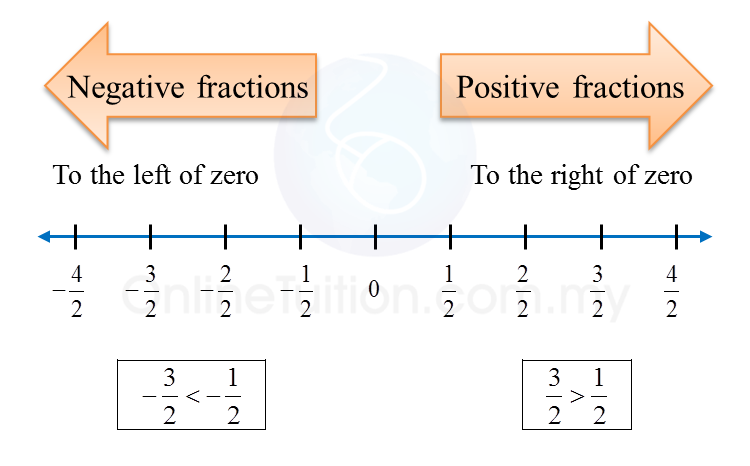# 1.2.2 Basic Arithmetic Operations Involving Integers

1.2.2a Fractions
1. Positive fractions are  fractions with the positive sign (+), and their values are greater than 0.
2. Negative fractions are  fractions with the negative sign (–), and their values are less than 0.1.2.2b Decimals
1. There are positive decimals and negative decimals.
2. Positive decimals are greater than zero and negative decimals are less than zero.1.2.2c Rational Numbers
1. All integers are rational numbers.
2.The computations of directed numbers is the same as that for whole numbers.

Example 2:
(a)  $-\frac{1}{2}+\left(-0.37\right)-\left(-5\right)$
(b)   [(–28) – (–4)] ÷ (–5.147 – 0.853)

Solution:
(a)
$\begin{array}{l}-\frac{1}{2}+\left(-0.37\right)-\left(-5\right)\\ =-0.5-0.37+5\\ =-0.87+5\\ =4.13\end{array}$

(b)
[(–28) – (–4)] ÷ (–5.147 – 0.853)
= [–28 + 4] ÷ (–6)
= (–24) ÷ (–6)
= 4